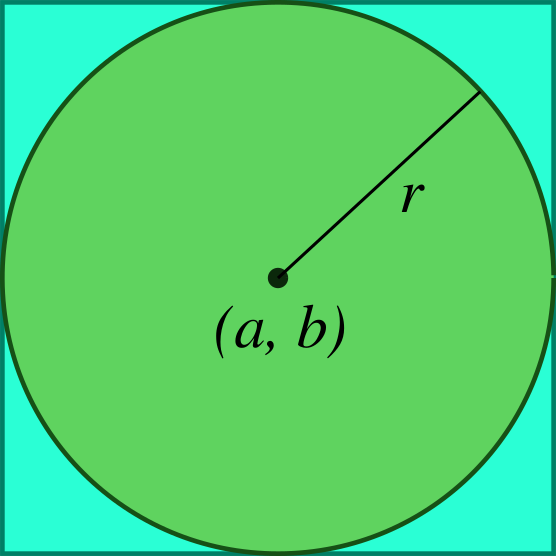# PHP - MySQL Geolocation: How to Retrieve Nearest Data from MySQL Using GPS coordinates

3729
Share:# Question

How to Retrieve Nearest Data from MySQL Using GPS coordinates

Location-based service is getting more popular these days. Hence, the most common query to database server is to find all data points that are within a given radius `r` from point `(a, b)`. The problem is that to calculate the radius from a point, we'll end up with famous Pythagoras theorem.

`SELECT row_id, x, y FROM some_table WHERE r >= sqrt((a-x)*(a-x)+(b-y)*(b-y));`

While this is a simple concept, actually the squares and square roots are too complex for MySQL to make any useful optimization on which index to use. It ends up calculating the radius for all records in the entire table, which could cause performance issue.

So, how could we optimize the use of MySQL index in this case?

Let's draw a circle.Let's draw a square outside the circle.I believe now we can see how we could write a query which doesn't scan for all records in database. Here's the detail:

Initial query to find all data points within a square that has point (a,b) at its center is:

`SELECT row_id, x, y FROM some_table WHERE ABS(x-a) < r AND ABS(y-b) < r; //parameters: a, r, b, r`

To make it easier for MySQL to use an index for this query is to adjust the query a little bit:

`SELECT row_id, x, y FROM some_table WHERE (x < r-a AND x > a-r) AND (y < r-b AND y > b-r);`

With the above query, MySQL can quickly return you all records that are within a certain square that has point (a,b) at its center.

Next, because what we need is a radius, we can combine with our first query:

`SELECT row_id, x, y FROM some_table WHERE (x < (a+r) AND x > (a-r)) AND (y < (b+r) AND y > (b-r)) AND r >= sqrt((a-x)*(a-x)+(b-y)*(b-y));`

Well, that's it. Now we have an effective query to find all data points within given radius and coordinate.

I have another related article, about how to calculate distance between two coordinates in PHP.

Tags
Share: